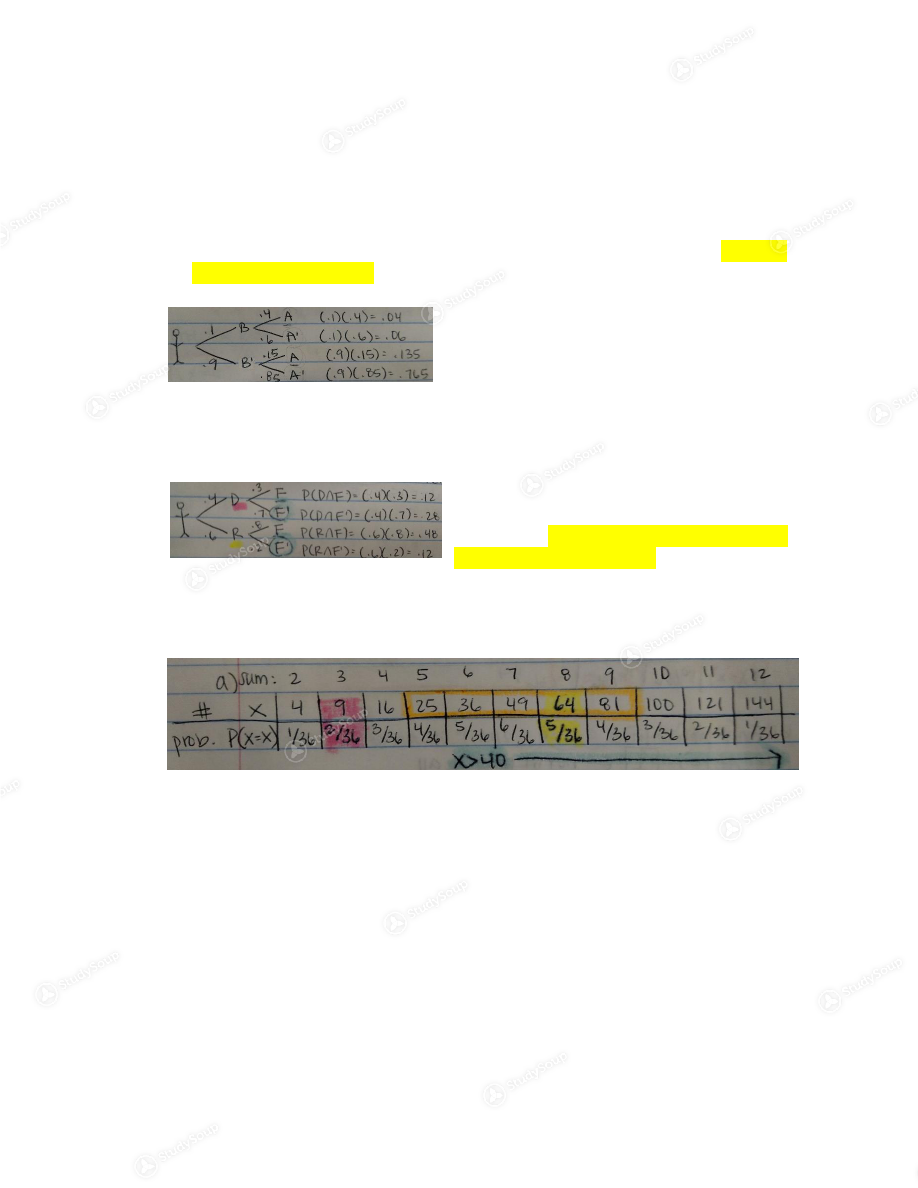×

# ASU - MAT 211 - Study Guide

### Created by: Ju Lee Elite Notetaker

Schools > Arizona State University > Math > MAT 211 > ASU - MAT 211 - Study Guide

ASU - MAT 211 - Study Guide

##### Description: This study guide covers chapters 6.3 & 6.4, 7.1 to 7.3, 7.4, 7.5, 7.6, 8.1, 8.2, 8.3, 8.4, 8.5, P.1, P.2 and P.3
0 5 3 59 Reviews
This preview shows pages 1 - 2 of a 4 page document. to view the rest of the contentMAT 211 Final Exam Study Guide- Abramson’s Class     **Final will be on chapters 6.3 & 6.4, 7.1 to 7.3, 7.4, 7.5, 7.6, 8.1, 8.2, 8.3, 8.4, 8.5, P.1, P.2 and P.3
1)  FCP, Permutations, Combinations, 7- letter sequence
(6.3 & 6.4)   a.  Ex FCP: 4 Appetizer, 10 main courses, 3 salads, 5 deserts. How many 3 course meals  are possible, order either appetizer or salad NOT both. (when there is “either, or” it
Answer:  (4 x 10 x 5) + (3 x 10 x 5) = 200+150= 350 course meals    b.  Ex Permutation: P(5,3) to order 5 objects 3 at a time. (On Calc: MathProb#2)  Answer:  P(5,3)= 5 x 4 x 3= 60    c.  Ex Combination: C(5,3) 5 objects, choose 3 at a time. (On Calc: MathProb#3)  Answer:  C(5,3)= (5x4x3)/(3x2x1)= 10    d.  Ex 7 letter strings: How many “strings” can be formed from “a,b,c,d,e,f,g”, no  repetition of letters.   Answer:   i.  7 letter string 7 x 6 x 5 x 4 x 3 x 2 x 1= 5,040 = P(7,7)  ii.  5 letter string 7 x 6 x 5 x 4 x 3= 2,520 = P(7,5)  iii.  7 letter strings, but “c” must be the 2 nd  letter 6 x 1 x 5 x 4 x 3 x 2 x 1= 720  iv.  7 letter strings, but “a” must come before “b”  7!/2= 2520    2)  Probability- marbles, lottery  (7.1-7.4)  and Independent Events word problems  (7.5)   a.  Ex Bag of Marbles  3 red, 4 green, 2 white, 3 pink; grab 7 marbles  Answer:  i.  P(all red marbles)= [C(3,3)*C(9,4)]/C(12,7)= 7/44  ii.  P( don’t have all the red marbles)= 1- P(opposite)= 1-(7/44)= 37/44    b.  Ex Lottery: Lottery number 0 to 30 (there is 31 numbers total) select 6 numbers  (order does not matter because as long as the numbers fill up 6 spaces), no
repetition.
Answer:  i.  P( all 6 #’s match)= n(s)/n(t)= 1/C(31,6)= 1/736,281  ii.  P( 5 of 6 #’s match)= [C(6,5)*C(25,1)]/C(31,6)= 150/736,281    c.  Ex Independent Events: P(A|B)= 0.4, P(B)= 0.6, P(A B)=?  Answer:  P(A|B)= P(A∩B)/P(B) 0.4= X/0.6  0.4(0.6)= 2.4    d.  Ex Independent Events Word problems: D= democrats and R= republicans; F= for  death penalty F’= against death penalty; P(R)= 0.6, P(F|D)= 0.7, P(F’|R)= 0.4Answer:  i.  P(F’|D)= 0.3  ii.  P(D∩F’)= 0.12  iii.  P(R∩F)= 0.36    3)  Bayes  (7.6) , Probability Distributions  (8.1) , Bernoulli  (8.2) , Z-scores  (8.5)   a.  Ex Bayes: P(A|B)= 0.4, P(B)= 0.1, P(A|B’)= 0.15. Find P(B|A) and P(B|A’). Formula:  given/possible outcomes  Answer:  P(B|A)= .04/(0.4+.135)= 8/35

P(B’|A’)=
.765/(.06+.765)= 51/55

b.  Ex Bayes word problem: P(Democrat)= 0.4, P(F|D)= 0.3, P(F|R)= 0.8. Find P(D|F),  P(R|F’), and P(F’|D).   Answer:  P(D|F)= .12/(.12+.48)= .12/.60= 1/5
P(R|F’)=
.12/(.28+.12)= .12/.40= 3/10
P(F’|D)= 0.7 (NOT Bayes Theorem just read
directly off of tree diagram)
c.  Ex Probability Distributions: Roll two die, x= square of sum of #’s on 2 die. Find the  probability.  Answer:
P(x=9)= 2/36
P(x=64)= 5/36
P(x=3)= 0
P(x>40)=
(6+5+4+3+2+1)/36= 21/36
P(25<= x<100)=
(4+5+6+5+4)/ 36= 24/36
P(x>.5)=1
P(x<3)= 0

d.  Ex Bernoulli Trials: Perform 5 Bernoulli Trials, p=0.2 Find x=2  Answer:  C(5,2) (0.2) 2  (0.8) 3 = 128/625

This is the end of the preview. Please to view the rest of the contentJoin more than 18,000+ college students at Arizona State University who use StudySoup to get ahead
4 Pages 405 Views 324 Unlocks
• Notes, Study Guides, Flashcards + More!Join more than 18,000+ college students at Arizona State University who use StudySoup to get ahead